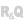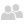Home |Sign in | EnglishHelpEditorsControls (window/page/report)ControlsSpreadsheet controlThe Spreadsheet control: Available formulasOverviewFormulas available in standardSee alsoEvents associated with a Spreadsheet controlHandling a Spreadsheet control by programming (in a report)Handling a Spreadsheet control by programming (in a window)Handling a Spreadsheet control by programming (in a window) (prefixed syntax)Printing the content of a Spreadsheet control (AAF)Properties associated with the Spreadsheet controlSpreadsheet control in a reportSpreadsheet control in a windowSpreadsheet: Using the ribbonThe Spreadsheet controlThe Spreadsheet control: Available formulas
• Overview
• Formulas available in standard
WINDEVWEBDEVWINDEV MobileOthersOverview
At run time, in the Spreadsheet control, the end user directly enters the calculation formulas. The cells used can be selected with the mouse.
The Spreadsheet control proposes the main calculation functions and operators: sum, subtraction, multiplication, division, percentages, mean, min-max, condition (IF), calculation and comparison operators, ...
Several WLanguage functions can be used in the formulas such as the mathematical functions, string functions, date functions, financial functions, ...
In the formulas, you have the ability to use the WLanguage procedures that have been created for this purpose in the application (SpreadsheetAllowProcedure). For example, the application can propose the "Shipping cost" procedure that calculates the amount according to advanced parameters: weight, volume, speed, destination country, ...
This procedure can even be used in the spreadsheet!
Formulas available in standard
The available functions are as follows: